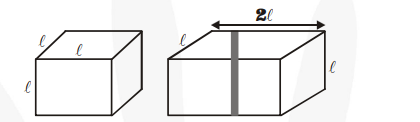# 2 cubes each of volume 64 cm`
Question:

2 cubes each of volume $64 \mathrm{~cm}^{3}$ are joined end to end. Find the surface area of the resulting cuboid.

Solution:

Let $\ell \mathrm{cm}$ be the length of an edge of the cube having volume $=64 \mathrm{~cm}^{3}$.

Then, $\ell^{3}=64=(4)^{3} \Rightarrow \ell=4 \mathrm{~cm}$

Now, the dimensions of the resulting cuboid made by joining two cubes (see figure) are 8 cm × 4 cm × 4 cm (i.e., length = 8 cm,

breadth $=4 \mathrm{~cm}$ and height $=4 \mathrm{~cm}$ )Surface area of cuboid $=2(\ell b+b h+h \ell)$

= 2 (8 × 4 + 4 × 4 + 4 × 8)

$=2(32+16+32)=2 \times 80=160 \mathrm{~cm}^{2}$